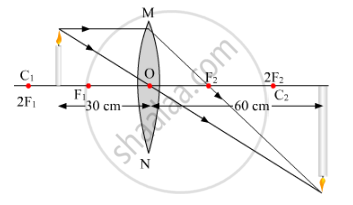# The Image of a Candle Flame Placed at a Distance of 30 Cm from a Spherical Lens is Formed on a Screen Placed on the Other Side of the Lens at a Distance of 60 Cm from the Optical Centre of the Lens. Identify the Type of Lens and Calculate Its Focal Length. If the Height of the Flame is 3 Cm, Find the Height of Its Image. - Science

The image of a candle flame placed at a distance of 30 cm from a spherical lens is formed on a screen placed on the other side of the lens at a distance of 60 cm from the optical centre of the lens. Identify the type of lens and calculate its focal length. If the height of the flame is 3 cm, find the height of its image.

#### SolutionSince the image is formed on the screen, the image is real. A concave lens cannot form a real image. Therefore, the lens is convex.

Focal length of the convex lens, f = ?

Object distance, u = ⇒f=20" role="presentation" style="position: relative;" data-mce-style="position: relative;">⇒f=2030 cm

Image distance, v = +60 cm

Since

1/v-1/u=1/f

therefore1/f=1/60-1/((-30))

rArr1/f=1/60+1/30

rArr1=((1+2))/60

rArr1/f=3/60

rArrf=20

Or

f = +20 cm

The magnification of convex lens, m=v/u

rArrm=60/-30

rArrm=-2

"Magnification, "m=h_i/h_o

where

hi  = Height of image

ho = Height of object

thereforem=h_i/3

rArrh_i=-2xx3

rArrh_i=-6

Therefore, height of image of candle flame is 6 cm.

Concept: Magnification Due to Spherical Lenses
Is there an error in this question or solution?
2014-2015 (March) Delhi Set 2

Share